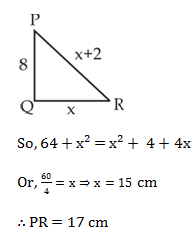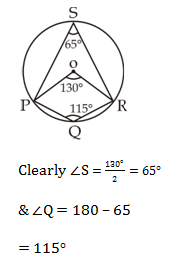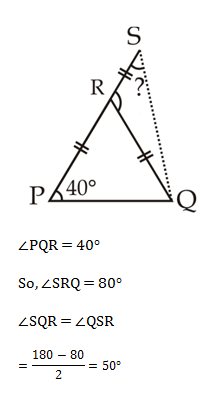# SSC Quantitative Aptitude Questions (Day-77)

Dear Aspirants, you can find the Quantitative Aptitude questions with detailed explanations for the SSC exams. Nowadays the competitive level of the exam has been increasing consistently. Due to the great demand for the government job, the level of the toughness reached greater. Candidates have to enhance the preparation process in order to drive in the right path. It doesn’t need to clear the prescribed cutoff. You must have to score good marks more than the cut off marks to get into the final provisional list. Here we have updating the Quantitative Aptitude questions with detailed explanations on a daily basis. You can practice with us and measure your level of preparation. According to that you can sculpt yourself in a proper way. SSC aspirants kindly make use of it and grab your success in your career.

Start Quiz

1)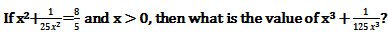(a) 7√2

(b) 5√2

(c) (7√2)/5

(d) 7√6

2) If ab (a +b)=1, then what is the value of
1/(a3 b3 )–a3–b3?

(a) –1

(b) 1

(c) 3

(d) –3

3) If y4 +
1/y4 = 223 and y > 1, then find the value of y2 +1/y2 ?

(a) 15

(b) 14

(c) 14.86

(d) 16

4) If (c – d) = (c + d)/5 = (cd)/3 and c, d ≠ 0 then what is the value of cd?

(a) 1/2

(b) 3/2

(c) 5/2

(d) 5/4

5)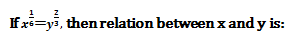(a) x = y2

(b) x = y4

(c) x4 = y

(d) x3 = y8

6) The distance between the centres of two circles of radius 9 cm and 6 cm is 17 cm. What is the length (in cm) of the transverse common tangent?

(a) 9

(b) 11

(c) 7

(d) 8

7) The length of the direct common tangent of two circles of radius 8 cm and 3 cm is 12 cm. What is the distance (in cm) between the centres of the circles?

(a) 15

(b) 13

(c) 14

(d) 17

8) ΔPQR is a right angled at Q. If PQ = 8 cm and PR = (QR + 2) cm. What is the value (in cm) of PR?

(a) 17

(b) 15

(c) 19

(d) 18

9) In the given figure, O is the center of the circle. If
POR = 130o, then what is the value (in degree) of S and Q respectively.

(a) 65, 115

(b) 55, 125

(c) 60, 120

(d) 65, 120

10)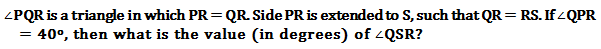(a) 45

(b) 50

(c) 55

(d) 41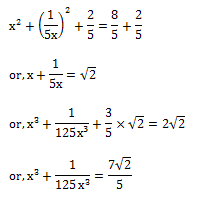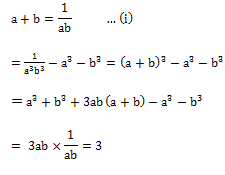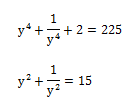c – d = k

c + d = 5k

∴ c = 3k

& d = 2k

But, cd/3 = k

or, 6k²/3 = k

Or k = 1/2,

So, cd = 3/2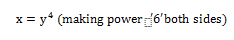TCT = √(289 – 225)

= 8 cm

DCT =√(d2 – 25)

144 + 25 = d²

Or, d = 13 cm